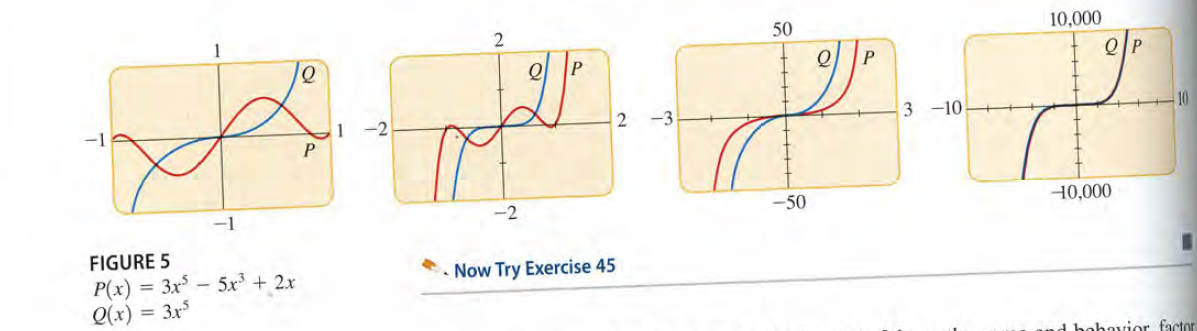# 50 10,000 2 -3 3 -10 10 -1 -2 -50 10,000 FIGURE 5 Now Try Exercise 45 P(x) = 3x – 5x³ + 2x Q(x) = 3x d bohavior factor

Question

Determine the end behaviour of P. Compare the graphs of P and in large and small viewing rectangles, as give.

P(x) = x4 - 7x2 + 5x + 5;  Q(x) = x4help_outlineImage Transcriptionclose50 10,000 2 -3 3 -10 10 -1 -2 -50 10,000 FIGURE 5 Now Try Exercise 45 P(x) = 3x – 5x³ + 2x Q(x) = 3x d bohavior factor fullscreen

### Want to see this answer and more?

Experts are waiting 24/7 to provide step-by-step solutions in as fast as 30 minutes!*

*Response times may vary by subject and question complexity. Median response time is 34 minutes for paid subscribers and may be longer for promotional offers.
Tagged in
Math
Algebra

### Equations and In-equations